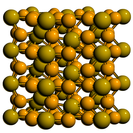Crystal Lattice Structures: Creation Date: 21 Oct 1995 Last Modified: 21 Oct 2004

# The Original Fe2P (C22) Crystal StructureYou can now

• This is the structure given in Strukturbericht Vol. II. As noted by Wyckoff, the structure, which was ``generally accepted for years, has recently been shown to be incorrect.'' (Vol I., p 360) This corrected structure, as given in Pearson's Handbook, is given in the revised Fe2P page.
• When z1 is set to zero this structure reverts to the revised Fe2P structure.
• We used the FINDSYM code to convert the Strukturbericht notation into the modern form.

• Prototype: Fe2P (original)
• Pearson Symbol: hP9
• Strukturbericht Designation: C22
• Space Group: P321 (Cartesian and lattice coordinate listings available)
• Number: 150
• Reference: Strukturbericht, Vol II, p. 15.
• Primitive Vectors:  A1 = ½ a X - ½ 3½ a Y A2 = ½ a X + ½ 3½ a Y A3 = c Z
• Basis Vectors:  B1 = ½ A3 = ½ c Z (P-I) (1b) B2 = 1/3 A1 + 2/3 A2 + z1 A3 = ½ a X + 12-½ a Y + z1 Z (P-II) (2d) B3 = 2/3 A1 + 1/3 A2 - z1 A3 = ½ a X - 12-½ a Y - z1 Z (P-II) (2d) B4 = x2 A1 = ½ x2 a X - ½ x2 3½ a Y (Fe-I) (3e) B5 = x2 A2 = ½ x2 a X + ½ x2 3½ a Y (Fe-I) (3e) B6 = - x2 A1 - x2 A2 = - x2 a X (Fe-I) (3e) B7 = x3 A1 + ½ A3 = ½ x3 a X - ½ x3 3½ a Y + ½ c Z (Fe-II) (3f) B8 = x3 A2 + ½ A3 = ½ x3 a X + ½ x3 3½ a Y + ½ c Z (Fe-II) (3f) B9 = - x3 A1 - x3 A2 + ½ A3 = - x3 a X + ½ c Z (Fe-II) (3f)

Go back to the hexagonal close-packed structures page.

Go back to the IIIb-VIIb Structures page.

Go back to Crystal Lattice Structure page.

 Structures indexed by: This is a mirror of an old page created at theNaval Research LaboratoryCenter for Computational Materials ScienceThe maintained successor is hosted at http://www.aflowlib.org/CrystalDatabase/ and published as M. Mehl et al., Comput. Mater. Sci. 136 (Supp.), S1-S828 (2017).